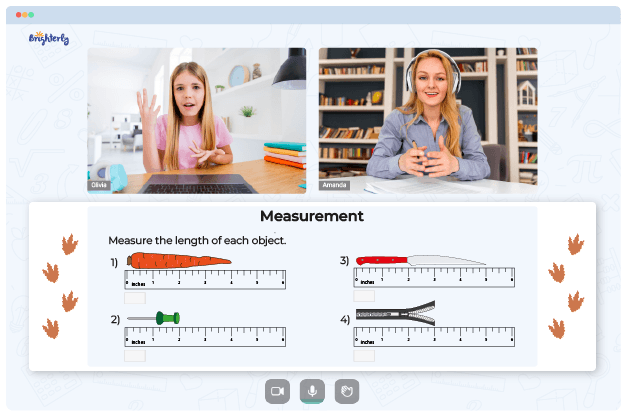# Metric System Worksheets

The metric system is based on the International System of Units (SI) and uses units such as meters, grams, and liters to measure length, mass, and volume. The metric system conversions worksheet helps kids understand the metric system as it is used in many everyday activities and professions. Kids can understand the metric system easier if they use free activities and examples available in the worksheets that are relevant and engaging tools.

## How It Works

To solve exercises from metric system conversions worksheets, children need to know the appropriate unit of measurement for the quantity they are measuring and the conversion factor for converting between units. For example, to measure the length of an object, they would use a meter stick or a ruler, and to convert length from meters to centimeters, they would multiply the length by 100, which is the conversion factor for meters to centimeters.

Math for Kids

Is Your Child Struggling With Math?
1:1 Online Math Tutoring## Benefits of the Metric System Worksheet

Working with the metric system conversion worksheet helps children understand and compare measurements in different contexts, such as determining the weight of an object or the temperature of a substance. Metric system conversions worksheet can also help children understand and apply mathematical concepts, such as conversions and calculations involving measurements.### Metric System Worksheets PDF

The Metric System Worksheet### Metric System Worksheets PDF

Metric System Worksheet### Metric System Worksheets PDF

Metric System Conversions Worksheets### Metric System Worksheets PDF

Metric System Conversion Worksheet

### More Measurement Worksheets

Need help with Measurement?• Does your child struggle to understand measurement?
• Try learning with an online tutor.

Is your child having difficulties with grasping the concept of measurement? An online tutor could provide the necessary help.